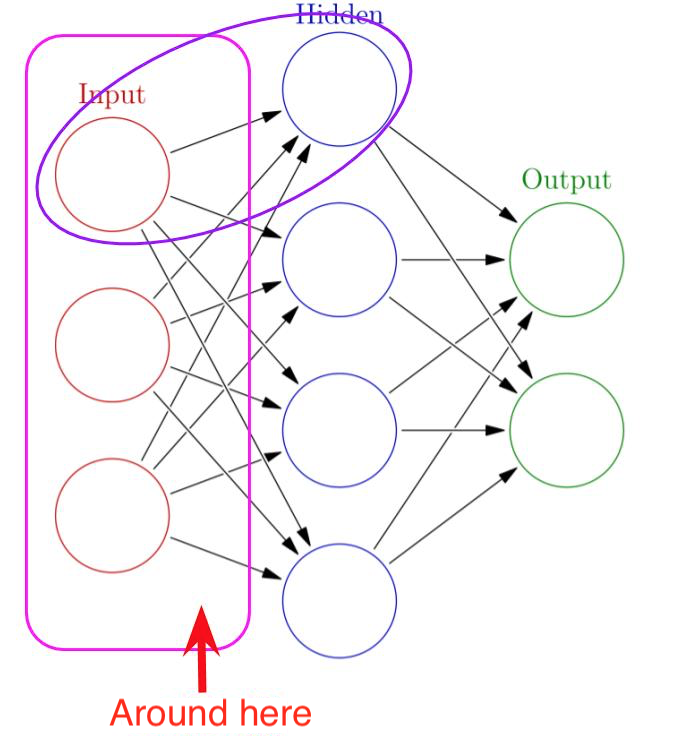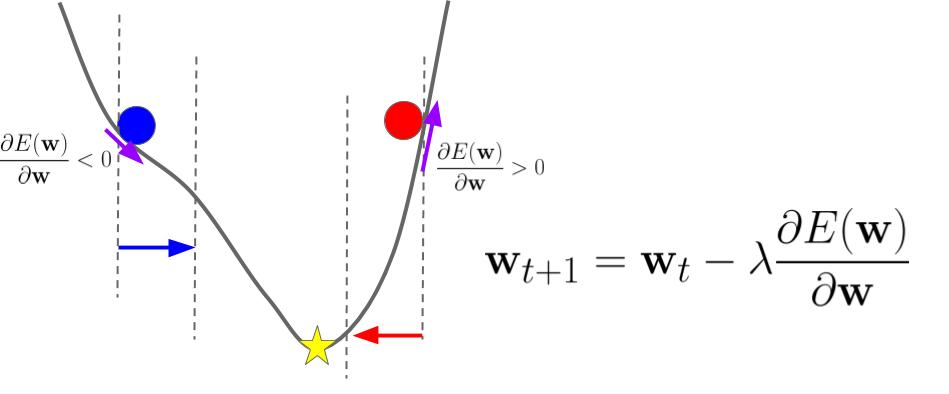# What kinds of mathematics are needed if you want to learn machine learning

This post is a reproduced version of the post in my Japanese blog.

For years, a lot of beginners in machine learning have asked me such as "Do I have to learn mathematics? What kind? To what extent?" and sometimes I've found it very hard to explain in a few words. Very fortunately, once I learned linear algebra and calculus when I was a student in an department of engineering so it's very familiar to me and useful for understanding theoretical aspects of machine learning.

But recently more and more beginners are rushing into machine learning or "AI" field to get more opportunity or even jobs. As far as I've seen, some of such people have never learned university-level mathematics although ML requires them. Very much unfortunately, most of them already graduated from their university years ago and they have little opportunity for learning mathematics in class. I think they need a kind of guidelines for learning mathematics for understanding machine learning.

In this post, I'd like to review a few kinds of mathematics that may be required for understanding modern machine learning, for such beginners. FYI I have one disclaimer: I'm never mathematical expert, so there might be incorrect or wrong points in terms of mathematics. If you see any points, don't hesitate to let me know!

### Overview: mathematics that enables you to understand what NN written by TensorFlow means

In my Japanese blog, I've written a series of posts about how to write NNs in TensorFlow.

When I wrote these ones, I felt: "Ah... this is very mmmmmmuch convenient and useful because what I have to do is just writing codes like maths seen in texts on Deep Learning and they should work as NN!!!" Yes, that's it for me :)

For example, let's see a typical code of DNN for MNIST classification. This is just what TensorFlow describes what a typical text of Deep Learning describes with maths.

x = tf.placeholder(tf.float32, [None, 784])

# 1st layer
## Define parameters
W1 = tf.Variable(tf.truncated_normal([784, 512], mean=0.0, stddev=tf.sqrt(2.0 / (784.0 + 512.0))))
## Define bias
b1 = tf.Variable(tf.zeros())
## Return prediction values (fitted values for training data) by matrix calculation
y1 = tf.matmul(x, W1) + b1
## Activation function (ReLU here)
y1 = tf.nn.relu(y1)

# 2nd layer
W2 = tf.Variable(tf.truncated_normal([512, 256], mean=0.0, stddev=tf.sqrt(2.0 / (512.0 + 256.0))))
b2 = tf.Variable(tf.zeros())
y2 = tf.matmul(y1, W2) + b2
y2 = tf.nn.relu(y2)

# Fully connceted
W3 = tf.Variable(tf.truncated_normal([256, 10], mean=0.0, stddev=tf.sqrt(2.0 / (256.0 + 10.0))))
b3 = tf.Variable(tf.zeros())
y3 = tf.matmul(y2, W3) + b3

# Optimize by gradient descent (momentum)
y = tf.placeholder(tf.int64, [None, 1])
y_ = tf.one_hot(indices = y, depth = 10)
global_step = tf.Variable(0, trainable=False)
starter_learning_rate = 0.001
learning_rate = tf.train.exponential_decay(starter_learning_rate, global_step,
10000, 1 - 1e-6, staircase=True)
cost = tf.reduce_mean(tf.nn.softmax_cross_entropy_with_logits(labels = y_, logits = y3))
optimizer = tf.train.MomentumOptimizer(learning_rate, momentum = 0.9, use_nesterov=True).minimize(cost, global_step = global_step)


Joke? No, no, I'm serious. That was the point that I've felt the most impressive when I wrote TensorFlow. For example, we can compose any kinds of NNs proposed by research articles with full of maths published on arXiv every day, just by using TensorFlow. What we only have to do is to simply follow all maths and description of networks by writing corresponding codes with TensorFlow*1.

So here I write down some points about to what extent mathematics are needed in order to write NNs with TensorFlow and to understand its meaning, from the viewpoint of me as data scientist without mathematics expertise.

### LInear algebra: you can understand what "tf.matmul" doesNeedless to say, each "layer" of NNN is almost equal to ordinary linear regression - linear summation of "parameter x independent variable". Then it means that a linear summation of a lot of "parameter x input" in a certain layer becomes a new input to the next layer. OK, let's write it down in linear algebra. With parameters, inputs, rowand columnof a dataset (independent variables), you can write as below.This means each of NN layers. But I think it's still messy. Using matrix multiplication of linear algebra, you can write as below.,,,Yes, this is very simple just as a formula - linear algebra can easily make it very simple as above. If you write it down with TensorFlow, it's also simple as the same as the expression above.

y1 = tf.matmul(x, W1) + b1


If you use "tf.matmul", the computation here is done by TensorFlow with giving only 3 data. What you have to do here is only to think how each layer of NN can be represented in linear algebra. Knowledge in linear algebra will make you easily implement NNs.

### Calculus: you can understand what tf.train.GradientDescentOptimizer(rate).minimize(cost) does when NN model seeks for its optimum of parameters

For NN, usually we use gradient descent in order to determine the best parameters for training data, with minimizing error (loss) function that represents error or loss between model prediction values and the training data. Strictly in NN, we have to run optimization with error back propagation with which it runs back in the network, but TensorFlow automatically computes it so we don't have to care about it. In general, an algorithm of gradient descent is described as follows.I think this formula itself says very well about the algorithm :) But it doesn't clearly tell why people usually argue about "vanishing gradient" for many kinds of Deep Learning.This is a figure in one of my past presentations. In short, "gradient" means "derivative" and "derivative" means "slope". It's my understanding that gradient descent uses "slope" given by a slight change of parameters in order to decrease error (loss) between model prediction value and the training data and to reach a star point in the figure above finally. Pay attention to a sign of derivative at each point; it means "direction" of "slope". This algorithm sophisticatedly makes each step move toward "valley".means a learning rate with which parameters should be changed against "slope" at that point.

For example, I wrote a simple TensorFlow code of a simple linear regression.

# Make a "layer" with matrix multiplication
x = tf.placeholder(tf.float32, [None, 13])
y = tf.placeholder(tf.float32, [None, 1])
W = tf.Variable(tf.zeros([13,1]))
b = tf.Variable(tf.zeros())

## First, define model prediction value
y_reg = tf.matmul(x, W) + b
## Error (loss) defined by the layer and the training data
cost = tf.losses.mean_squared_error(labels = y, predictions = y_reg)
## Give a learning rate
rate = 0.1
## Determine the best parameters by gradient descent with error (loss) and learning rate


At the last row, you see "tf.train.GradientDescentOptimizer(rate).minimize(cost)" - this is the method of TensorFlow that minimizes error (loss) by gradient descent. You can get "gradient" by "derivative" and you can use "gradient" as "slope" to seek for the optimal parameters with this method. This is why you should learn even an entry level of calculus to understand what's going on behind NN.

### Misc: you should learnat least

Of course you should know whatis to understand what "tf.nn.softmax" does.

y = tf.nn.softmax(tf.matmul(x, W) + b)


Why? It's because as typical texts on Deep Learning say that it meansas you know, it's softmax. :) Am I joking? No, no, I mean that not a few beginners may forget even. In Japan, it's not university-level, but high school-level maths... if you don't remember, you should learn again in order to understand what softmax is.

In addition, it would be nice if we can represent every mathematical expression by programming. Although it's written in Japanese, but this slideshare by @ must be helpful if you want to learn it. This is a great document I think.

### Summary

Of course I know that there are a lot of cases in which even maths mentioned above are not enough. For example, it was really not enough when I tried to read and understand the original paper about HSIC... so I mean that we have to learn maths more and more, as much as possible we can. In particular, if you want to read through research articles on machine learning updated onto arXiv or top-conference papers such as NIPS / KDD / ICLR / AAAI / ICML / ACL etc., you have to learn maths in further advanced level.

However, if you are just a "user" of machine learning techniques which are implemented as OSS, you don't need maths. If you want to implement SOTA NNs with reading the latest research papers, you need the maths mentioned above.

Personally, I remember one thing: when I once tried to implement non-linear kernel SVM and its sequential minimal optimization*2, I had to write a little complicated logic and implement Lagrange multiplier by hand. I think that such a kind of maths may be required when we learn machine learning.

*1:Oops, still I've missed writing GANs from scratch...

*2:At that time I wrote it with Matlab because it was just a little after I joined the current industry